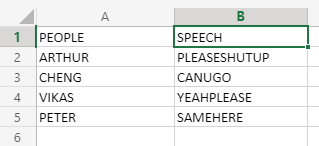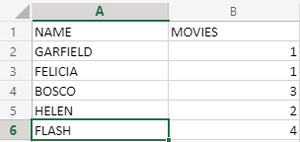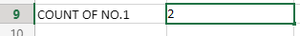# Why Two Minus Signs (–) in an Excel Formula?

• Last Updated : 04 Aug, 2021

If you start studying advanced EXCEL, you must have noticed that many formulas, even the most basic formulas, when used with other formulas use this “–” symbol, and what we observe after applying these formulas, is that instead of getting TRUE value, we get output as 1, whereas if we are expecting FALSE value, we are getting output as 0.

So, you must have noticed that the “–” operator(Double negation operator)behaves like a binary operator in this. Yes, this operator can contrast the whole result in form of numbers.

Attention reader! Don’t stop learning now. If you are an Excel beginner (or an intermediate) and want to learn Excel, Geeksforgeeks brings the perfect course for you to start, Diving Into Excel

First, we will see some examples which use this concept.

### 1.Using LEN Function:

Here, we will be having data of  4 students, and whoever speaks more than 10 characters, we will target him/her for harassment, by counting that student. The dataset will look like this:Now, applying a simple formula of function LEN, we will get our desired result. The formula will be:

`=LEN(B2:B5) > 10`

Now, as discussed, we will see what this function will do without any external changes in its syntax. It is going to generate a boolean “logical” value array of the given ranges, and as per the given condition. So, let’s see what that array will look like:

`={TRUE;FALSE;FALSE;FALSE}`

Now, as clear from the above-returned array, that only the first student is harassing according to the given condition.

Now, let’s suppose this array has to be sent to superiors for checking students data, and they don’t really accept boolean logical values as they will compute these array values in terms of numbers. So, that is a problem!!. So this is where “–” comes into play. Now, applying the same formula on the same dataset, with a little adjustment, the formula will look like this:

`=--(LEN(B2:B5)>10)`

Now, the modified array will look like:

`={1;0;0;0}`

Now, this array can be used to compute mathematical forms in a direct fashion.

### 2.Using SUMPRODUCT

Now, let’s suppose we have a database of people watching a particular movie and an excel file associated with it. We have an approximate guess that movie number 1 is very popular so most people will watch that movie only. So we want to know how many people watch movie number 1. The dataset will be:Now applying a simple formula to know the amount of 1’s in the MOVIES column. The formula will look like–

`=(\$B2:\$B6=1)`

But as we know we can’t really deal with boolean logical values in the computational part, so this formula is insufficient. Here we will use the “–” operator to resolve this. Now, applying this operator in the previous formula, the formula will look like–

`=--(\$B2:\$B6=1)`

Now, the array will look like:

`{1;1;0;0;0}`

Now we will directly apply the SUMPRODUCT function on this, to sum up, the truth values in the array. So the formula will be:

`=SUMPRODUCT(--(\$B2:\$B6=1))`

So we will get output as 2 in this dataset for this condition.So you see the importance of double negation operator in some cases. But in practical life, this operator is very powerful, as it can help you to compute in numeric form.

My Personal Notes arrow_drop_up